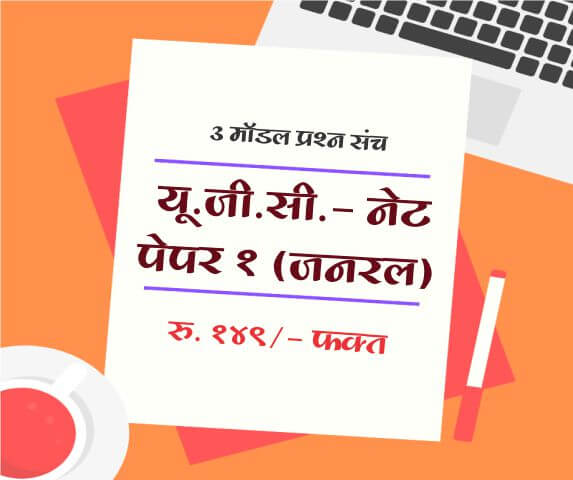# SIMPLE INTEREST - (ENGLISH)

Share Now

Simple Interest in Quantitative Aptitude Test

A simple interest in online aptitude test is an important topic of interest that falls in the category of Data sufficiency and Arithmetic section. While solving these questions, students often get confused among a few basic terms, and it hampers the accuracy of results. In such situations, it is better to learn some trusted tips and tricks that can ensure the proper solution for simple interest questions asked in bank exams and other aptitude tests.

In this article, we are going to study some basic terms related to the simple interest that can help you improve your knowledge base. If you are also preparing for a career aptitude test, it is better to go through the details below.

Simple Interest:

It is usually represented by SI in mathematical terms and can be defined as: When the interest on the sum that is borrowed for a specific duration of time is calculated uniformly, this terminology is better known as simple interest.

What is the Principal?

“Principal” in quantitative aptitude questions are also defined as “sum,” and it is the total money borrowed by a person for the specific duration of time. While solving the aptitude questions, it is generally represented by P.

What is the amount?

The addition of Principle and Simple Interest is better defined as Amount. In mathematical terms, it can be denoted as A = S.I. + P (Principle).

Interest:

Interest is defined as the extra money that is paid by the borrower to the lender as compensation for using his money for the specific duration of time. It is generally calculated on the Principle.

Time:

Time can be defined as the total duration for which person borrows or lends money.

Rate of Interest:

When the person borrows some amount, the rate of interest is defined as the interest that is charged by the lender to the borrower on principle for the set duration.

Per annum:

While going through Simple Interest questions, you will often read a statement saying, “Rate of interest R% per annum.” It represents interest on the sum for one year. In case if it is not stated separately, then the rate of interest is generally assumed to be on an annual basis.

Important formula:

(Simple Interest) S.I = [(Principle X Rate of interest per annum X Time) / (100)]

From this formula; we can define these associated terms as:

(Principle) P = (100 X Simple Interest) / (Rate of interest X Time)

(Rate of Interest) R = (100 X Simple Interest) / (Principle x Time)

(Time) T = (100 x Simple Interest) / (Principle x Rate of Interest)

Once you know the meaning of all these terms and remember formulas to calculate them, it becomes easier to find solutions for the questions given in the Quantitative Aptitude test. These concepts are more useful in the banking sector, so if you are planning to appear in an exam in the world of economics or banking, it is better to practice these questions to ensure higher accuracy.

#### Who are all can get the benefits from this QUANTITATIVE APTITUDE Question and Answers section?

Candidates preparing for following competitive exams can utilize Aptitude section to improve their skills.

• Bank Competitive Exam
• MPSC Competitive Exams
• SSC Competitive Exams
• L.I.C/ G. I.C Competitive Exams
• Railway Competitive Exam
• University Grants Commission (UGC)
• NET/ SET Competitive Exam
• Common Aptitude Test (CAT)
• Career Aptitude Test (IT Companies)

#### SIMPLE INTEREST - (ENGLISH) is useful for following exam preparation

 Quantitative Aptitude for Competitive Exam IBPS Bank Exam Maharashtra B.Ed. CET AP LAWCET TSLAWCET AILET IBPS RRB MPSC Sales tax Inspector UPTET RBI Assistant SBI Clerk MPSC State Service MPSC: Assistant Motor Vehicle Inspector Examination MPSC State Excise Sub-Inspector CLAT SSC-CGL MBA Entrance MPSC Competitive Exams Bank Aptitude Test SSC GD (Constable) Recruitment

#### Mock Exam Papers

Latest Articles
Popular Articles
Upcoming Exam Forms

Full Length Mock Tests
Timer Based Exams
Instant Result and assesment
Detailed analasys of Result##### UGC NET SET Paper 1 Mock Tests

UGC-NET / SET Paper 1 mock test

3 Mock Tests, 150 Questions with Explanations

Rs: 149 Only/-##### LAW MH-CET 2019 (3 YEAR COURSE)

Mock test for MH CET Law 2019 for three year law programme

3 Mock Tests, 450 Questions with Explanations

Rs: 249 Only/-##### यू. जी. सी. - नेट पेपर १ (जनरल)

यू. जी. सी. - नेट पेपर १ (अनिवार्य) सामान्य प्रश्न हिंदीमें

3 Mock Tests, 150 Questions with Explanations

Rs: 149 Only/-